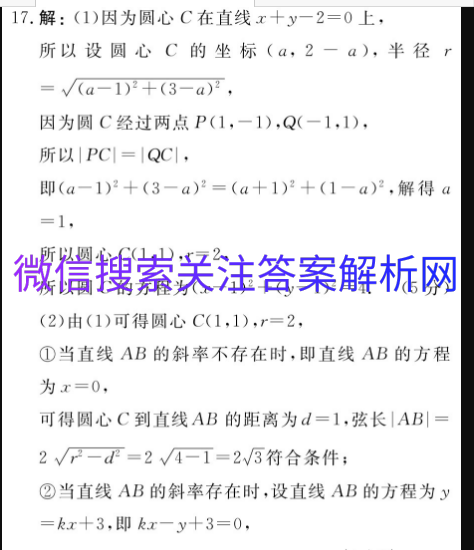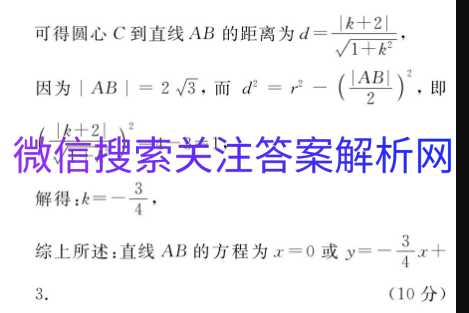# 炎德英才大联考2021 理数

2021-01-09 21:5517.解:(1)因为圆心C在直线x+y-2=0上,所以设圆心C的坐标(a,2-a),半径r=√(a-1)2+(3-a)2,因为圆C经过两点P(1,-1),Q(-1,1),所以PC=QC|即(a-1)2+(3-a)2=(a+1)2+(1-a)2,解得a=1,所以圆心C(1,1),r=2,所以圆C的方程为(x-1)2+(y-1)2=4.(5分)(2)由(1)可得圆心C(1,1),r=2,①当直线AB的斜率不存在时,即直线AB的方程为x=0,可得圆心C到直线AB的距离为d=1,弦长|AB|=2√-d2=2√4-1=23符合条件;②当直线AB的斜率存在时,设直线AB的方程为y=kx+3,即kx-y+3=0,可得圆心C到直线AB的距离为d=k+2√1+k2因为AB|=2√3,而d2=r2-(AB)22,即k+22√1+k=4-3=1,解得:k=-4综上所述:直线AB的方程为x=0或y=-x+3.(10分)